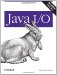# Byte Array Streams

It's sometimes convenient to use stream methods to manipulate data in byte arrays. For example, you might receive an array of raw bytes that you want to interpret as double-precision, floating-point numbers. (This is common when using UDP to transfer data across the Internet, for example.) The quickest way to do this is to use a DataInputStream. However, before you can create a data input stream, you first need to create a raw, byte-oriented stream. This is what the java.io.ByteArrayInputStream class gives you. Similarly, you might want to send a group of double-precision, floating-point numbers across the network with UDP. Before you can do this, you have to convert the numbers into bytes. The simplest solution is to use a data output stream chained to a java.io.ByteArrayOutputStream. By chaining the data output stream to a byte array output stream, you can write the binary form of the floating-point numbers into a byte array, then send the entire array in a single packet.

9.2.1. Byte Array Input Streams

The ByteArrayInputStream class reads data from a byte array using the methods of java.io.InputStream:

```public class ByteArrayInputStream extends InputStream
```

ByteArrayInputStream( ) has two constructors. Both take a byte array as an argument. This byte array is the buffer from which data will be read. The first constructor uses the entire buffer array as an input stream. The second constructor uses only the subarray of length bytes of buffer starting with the byte at offset.

```public ByteArrayInputStream(byte[] buffer)
public ByteArrayInputStream(byte[] buffer, int offset, int length)
```

Other than these two constructors, the ByteArrayInputStream class just has the usual read( ), available( ), close( ), mark( ), and reset( ) methods. Byte array input streams do support marking and resetting up to the full length of the stream. This is relatively straightforward to implement because, at any time, a byte array contains in memory all of the data in the stream. Unlike other kinds of streams, you don't have to worry that you'll try to reset further back than the buffer allows.

9.2.2. Byte Array Output Streams

The ByteArrayOutputStream class writes data into the successive components of a byte array using the methods of java.io.OutputStream:

```public class ByteArrayOutputStream extends OutputStream
```

This class has the following two constructors, plus the usual write( ), close( ), and flush( ) methods:

```public ByteArrayOutputStream( )
public ByteArrayOutputStream(int size)
```

The no-argument constructor uses a buffer of 32 bytes. The second constructor uses a user-specified buffer size. However, regardless of the initial size, the byte array output stream will expand its buffer as necessary to accommodate additional data.

To return the byte array that contains the written data, use the toByteArray( ) method:

```public byte[] toByteArray( )
```

There are also toString( ) methods that convert the bytes into a string. The no-argument version uses the platform's default encoding. The second method allows you to specify the encoding to be used:

```public String toString( )
public String toString(String encoding) throws UnsupportedEncodingException
```

Another common use of ByteArrayOutputStream is to accumulate data into an internal buffer and then quickly write the entire buffer onto another stream.

The writeTo( ) performs this task:

```public void writeTo(OutputStream out) throws IOException
```

Examples 9-2 uses a byte array output stream to implement a simple form of buffering. An array is created to hold the first n Fibonacci numbers in binary form, where n is specified on the command line. (The Fibonacci numbers are the sequence 1, 1, 2, 3, 5, 8, 13, and so on, where each number is calculated by adding the previous two numbers in the sequence.) The array is filled using the methods of java.io.DataOutputStream. Once the array is created, a file is opened and the data in the array is written into the file. Then the file is closed. This way, the data can be written quickly without requiring the file to be open while the program is calculating.

Example 9-2. The FibonacciFile program

 ```import java.io.*; public class FibonacciFile { public static void main(String args[]) throws IOException { int howMany = 20; // To avoid resizing the buffer, calculate the size of the // byte array in advance. ByteArrayOutputStream bout = new ByteArrayOutputStream(howMany*4); DataOutputStream dout = new DataOutputStream(bout); // First two Fibonacci numbers must be given // to start the process. int f1 = 1; int f2 = 1; dout.writeInt(f1); dout.writeInt(f2); // Now calculate the rest. for (int i = 3; i <= 20; i++) { int temp = f2; f2 = f2 + f1; f1 = temp; dout.writeInt(f2); } FileOutputStream fout = new FileOutputStream("fibonacci.dat"); try { bout.writeTo(fout); fout.flush( ); } finally { fout.close( ); } } } ```

You can use the FileDumper3 program from the previous chapter with the -i option to view the output. For example:

```\$ java FibonacciFile fibonacci.dat
\$ java FileDumper3 -i fibonacci.dat
1
1
2
3
5
8
13
21
34
55
...
```Java I/O
ISBN: 0596527500
EAN: 2147483647
Year: 2004
Pages: 244